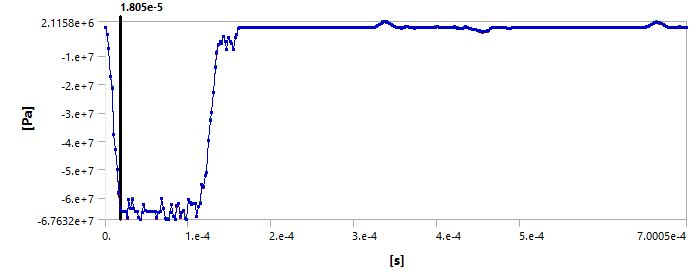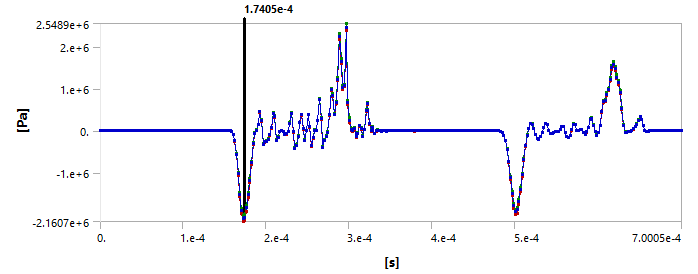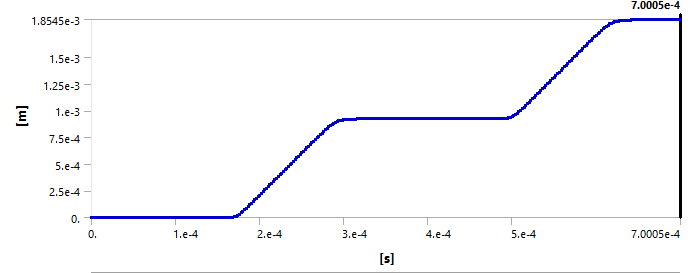## LS Dyna

Topics relate to LS-DYNA, Autodyn, Explicit STR and more

•aman7
Subscriber
Hello,nI am trying to simulate Split-Hopkinson Pressure bar using Ansys Explicit. I have designed the model except the striker bar. For the striker effect I am applying the pressure to the incident bar face equivalent to incident strain recorded during actual experiment as the bars will be elastic. Now after completion of simulation, I am adding User Defined Result Expression : SOUNDSPEED. The result is 6108 m/s. nIs it the same stress wave velocity?nIf Yes, then stress wave velocity in Al6061-T6 is around 5100 m/s. How to get accurate value?n
•peteroznewman
Subscriber
nPlease reply with a Pressure-Time graph of the striker effect. What is the time of the peak?nCreate and plot the Directional Deformation-Time result on the far face of the bar,nYou might need to filter the data if it is noisy.nCalculate the deltaT between the peak pressure and peak deformation.nYou know the length of the bar, L.nVelocity = L/deltaT.n
•Erik Kostson
Ansys Employee
What Peter said is good.nnWe just need to remember there is a phase speed and group speed, and if the stress waves are dispersive they are not the same. And there are different type of guided waves. You are likely looking at the longitudinal mode in a bar.nnSo what Peter described is the group velocity of a wave, and that should be what you need to calculate. nSo look at two points/locations along the bar (distance L between them), get their arrival times (so when the stress wave arrives) call it time_point 1 (point1 is closest to excitation), and time_point2, and then the (group) wave speed is L/(time_point2-time_point1). (time_point2>time_point1)nnHope this helpsnnErikn
•aman7
Subscriber
nThank You for reply. nI have attached the stress on the loading face and on the far face of bar.nInput stress is:nOn far face:nDirectional Deformation is:nNow I can understand your concept. If I consider the stress at loading face has reached at the far face in some time T = 1.74e-4 - 1.805e-5 = 1.56e-4 = 156 us (IS THIS CORRECT?)nThe length of bar = 1 m. thus velocity is 1/156e-6 s = 6410 m/snBut this is Al6061-T6 bar, for this wave velocity is (E/rho)^(1/2) = 5018. nThe user defined result of SOUNDSPEED is 6100 m/s.nhow can i get the correct value? Is Stress wave speed same as SOUNDSPEED?nThank You.n
•Erik Kostson
Ansys Employee
In the equation for the ((E/rho)^(1/2)), one does not account for Poisson's effect which is important in longitudinal waves in 3D bars or other 3D wave-guides and in 3D elasticity in general.nnThe equation we need to use for the speed needs to account for Poisson's and is thus also valid for 3D elasticity.nnFor AL6061 alu:nn=SQRT((68000000000*(1-0.33))/(2700*(1+0.33)*(1-2*0.33))) = 6109 m/s which is as the user defined results (SOUNDSPEED) from the explicit software.nnAll the bestnnEriknn
•aman7
Subscriber